NCERT Exemplar Solutions: Motion and Measurement of Distances

# NCERT Exemplar Solutions: Motion and Measurement of Distances - Science Class 6

### Multiple Choice Questions

Q.1. The distance between Delhi and Mumbai is usually expressed in units of
(a) decametre
(b) metre
(c) centimetre
(d) kilometre
Ans:
d
Solution:
The distance between Delhi and Mumbai is usually expressed in units of kilometre.

Q.2. Which of the following does not express a time interval?
(a) A day
(b) A second
(c) A school period
(d) Time of the first bell in the school
Ans:
d
Solution:
Time of the first bell in the school represents a point of time, not a time interval.

Q.3. Figure shows a measuring scale which is usually supplied with a geometry box. Which of the following distance cannot be measured with this scale by using it only once?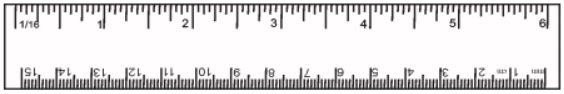(a) 0.1m
(b) 0.15 m
(c) 0.2 m
(d) 0.05 m
Ans: c

Solution: This scale is 0.15 m long. 0.2 m cannot be measured, if used only once.

Q.4. A piece of ribbon folded five times is placed along a 30 cm long measuring scale as shown in figure.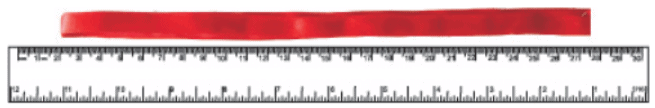The length of the ribbon is between
(a) 1.15 m – 1.25 m
(b) 1.25 m – 1.35 m
(c) 1.50 m – 1.60 m
(d) 1.60 m – 1.70 m.
Ans:
b
Solution: According to the above figure, the five times folded ribbon piece is (27.5 – 2) cm × 5 = 1.275 m long approximately.

Q.5. Paheli moves on a straight road from point A to point C. She takes 20 minutes to cover a certain distance AB and 30 minutes to cover the rest of distance BC. She then turns back and takes 30 minutes to cover the distance CB and 20 minutes to cover the rest of the distance to her starting point. She makes 5 rounds on the road the same way. Paheli concludes that her motion is
(a) only rectilinear motion
(b) only periodic motion
(c) rectilinear and periodic both
(d) neither rectilinear nor periodic
Ans:
c
Solution: Paheli is moving along a straight line with a fixed time period. So, her motion is rectilinear and periodic both.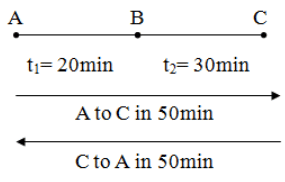Q.6. Bholu and Golu are playing in a ground. They start running from the same point A in the ground and reach point B at the same time by following the paths marked 1 and 2 respectively as shown in figure. Which of the following is/are true for the given situation?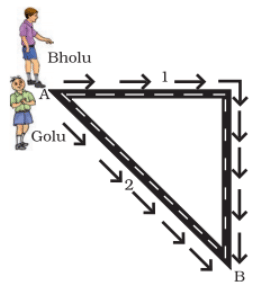As compared to Golu, Bholu covers a
(a) longer distance but with a lower speed
(b) longer distance with a higher speed
(c) shorter distance with a lower speed
(d) shorter distance with a higher speed
Ans:
b
Solution: Path 1 is longer than path 2. But Golu and Bholu both have reached point B at the same time, so Bholu’s speed is more than Golu’s speed.

Q.7. Four pieces of wooden sticks A, B, C and D are placed along the length of 30 cm long scale as shown in figure. Which one of them is 3.4 cm in length?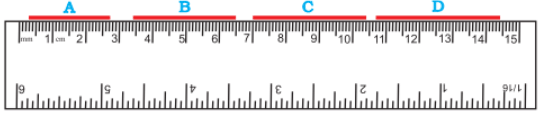(a) A
(b) B
(c) C
(d) D
Ans: c

Solution: We can observe from the given figure, that stick C lies between 10.4 cm and 7 cm. So, its length is (10.4 – 7) cm = 3.4 cm.

Q.8. Which of the following figure shows the correct placement of a block along a scale for measuring its length?
(a)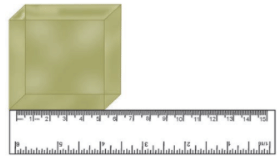(b)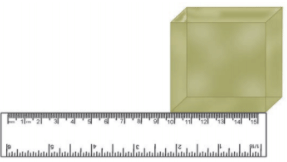(c)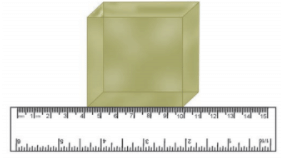(d)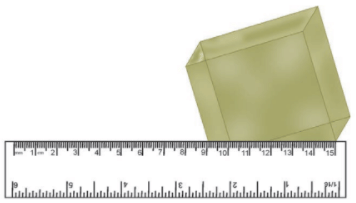Ans:
c
Solution:
Object should be placed on a scale such that its ends lie in between the readings marked on the scale.

Q.9. You are provided three scales A, B and C as shown in figure to measure a length of 10 cm.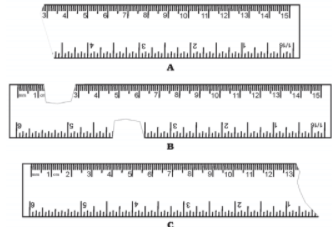For the correct measurement of the length you will use the scale

(a) A only
(b) B only
(c) C only
(d) Any of the three Scales.
Ans: d
Solution: Length of 10 cm is available in all the three scales A, B and C. So, any of the three scales’ can be used.

Q.10. Correct the following:
(i) The motion of a swing is an example of rectilinear motion.
(ii) 1m = 1000cm
Ans:
(i) No, the motion of a swing is not an example of rectilinear motion. The motion of a swing is an example of periodic motion as it repeats its motion after a fixed interval of time.
(ii) 1m = 100 cm. Each metre (m) is divided into 100 equal divisions, called centimetre (cm).

Q.11. Fill in the blanks.
(i) Motion of an object or a part of it around a fixed point is known as ______ motion.(ii) A body repeating its motion after certain interval of time is in ______ motion.
(iii) In rectilinear motion, object moves a ______ line ______ .
(iv) SI unit of length is ______ .
Ans: (i) Circular
(ii) periodic
(iii) along; straight
(iv) metre

Q.12. Write one example for each of the following type of motion.
(i) Rectilinear
(ii) Circular
(iii) Periodic
(iv) Circular and periodic
Ans:
(i) Rectilinear – An apple falling vertically downwards.
(ii) Circular – The motion of a point marked on the hands of a clock.
(iii) Periodic – Motion of a child on a swing.
(iv) Circular and periodic – Blades of a rotating fan.

Q.13.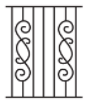The photograph given in figure shows a section of a grille made up of straight and curved iron bars. How would you measure the length of the bars of this section, so that the payment could be made to the contractor?
Ans:
As the grille is made up of straight and curved iron bars, so the length of the curved bars can be measured with the help of thread first and then can be further measured using a scale. The straight bars can be measured with measuring tape.

Q.14. Identify the different types of motion in the following word diagram given.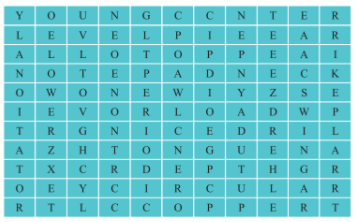Ans: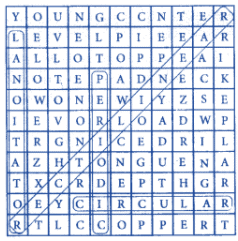Q.15. Four children measure the length of a table which was about 2m. Each of them used different ways to measure it.
(i) Sam measured it with a half metre long thread.
(ii) With a 15cm scale from her geometry box.
(iii) Reena measured it using her hand span.
(iv) Salim measured it using a 5m long measuring tape.
Which one of them would get the most accurate length? Give reason for your answer.
Ans:
(iv) Salim measured it using a 5 m long measuring tape.
By using a 5 m long measuring tape which is longer than the table, Salim would get the most accurate length. So, he can measure the length of the table in one go accurately.

Q.16. Match the events related to motion in column I with the types of motions given in column II.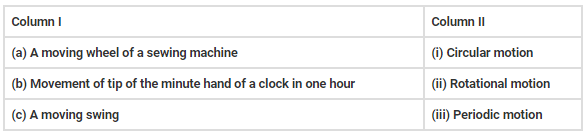Ans:
Here is the correct match: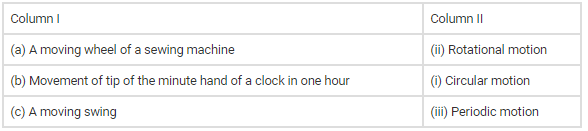Q.17. While travelling in a train, it appears that the trees near the track are moving whereas co-passengers appear to be stationary. Explain the reason.
Ans:
While sitting in a moving train, the trees appear to be moving in the opposite direction because their position is changing with respect to us. While the position of co-passengers appears to be in a stationary position since they are not changing with respect to us.

Q.18. How are the motions of a wheel of a moving bicycle and a mark on the blade of a moving electric fan different? Explain.
Ans:
The wheels of a moving bicycle indicate circular as well as rectilinear motion.
Wheels of bicycle rotate on the ground which in turn moves the bicycle forward.
A blade of a moving electric fan shows only circular motion as it is not moving from one place to another.

Q.19. Three students measured the length of a corridor and reported their measurements. The values of their measurements were different. What could be the reason for difference in their measurements? (Mention any three)
Ans: Some reasons for the difference in their measurement could be
(i) The measurement may not be along the shortest length in all three cases.
(ii) The measuring devices may not be standard.
(iii) Different measuring devices were used.
(iv) The end of the corridor may not be easily accessible.
(v) The smallest length that could be measured by different devices may be different.

Q.20. Boojho was riding in his bicycle along a straight road. He classified the motion of various parts of the bicycle as
(i) rectilinear motion
(ii) circular motion and
(iii) both rectilinear as well as circular motion. Can you list one part of the bicycle for each type of motion? Support your answer with reason.
Ans:
(i) Rectilinear motion: Handle of the bicycle has rectilinear motion. It moves in a straight line as the wheels of bicycle move forward.
(ii) Circular motion: Pedal of the bicycle is having circular motion it rotates with its shaft but does not move from its place.
(iii) Rectilinear and circular motion: Wheel of the moving bicycle is having both circular and rectilinear motion. Wheels rotate on their shafts as well as move forward on the ground.

The document NCERT Exemplar Solutions: Motion and Measurement of Distances | Science Class 6 is a part of the Class 6 Course Science Class 6.
All you need of Class 6 at this link: Class 6

## Science Class 6

73 videos|173 docs|37 tests

## FAQs on NCERT Exemplar Solutions: Motion and Measurement of Distances - Science Class 6

 1. What is motion?Ans. Motion is the change in position of an object with respect to its surroundings over time. It can be described in terms of distance, displacement, speed, velocity, and acceleration.
 2. What is the difference between distance and displacement?Ans. Distance refers to the total length covered by an object in its motion, irrespective of its direction. Displacement, on the other hand, is the shortest distance between the initial and final positions of the object in a particular direction.
 3. How is speed different from velocity?Ans. Speed is a scalar quantity that measures how fast an object is moving, whereas velocity is a vector quantity that measures both the speed and direction of an object's motion.
 4. What are the different units used to measure distance and speed?Ans. Distance is commonly measured in units like meters (m), kilometers (km), and centimeters (cm). Speed is usually measured in units like meters per second (m/s), kilometers per hour (km/h), and miles per hour (mph).
 5. How can we calculate the average speed of an object?Ans. The average speed of an object can be calculated by dividing the total distance covered by the object by the total time taken to cover that distance. The formula for average speed is: Average Speed = Total Distance / Total Time.

## Science Class 6

73 videos|173 docs|37 testsExplore Courses for Class 6 examSignup to see your scores go up within 7 days! Learn & Practice with 1000+ FREE Notes, Videos & Tests.
10M+ students study on EduRev
Track your progress, build streaks, highlight & save important lessons and more!
Related Searches

,

,

,

,

,

,

,

,

,

,

,

,

,

,

,

,

,

,

,

,

,

;